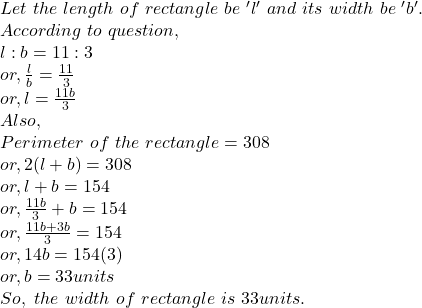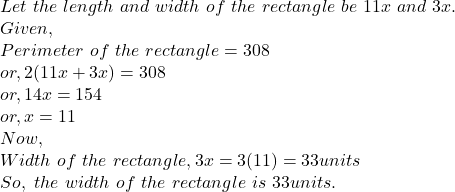## The ratio of length to width on a rectangle is 11:3. The perimeter of the figure is 308. what is the width of the rectangle

Question

The ratio of length to width on a rectangle is
11:3. The perimeter of the figure is 308. what is the
width of the rectangle

in progress 0
7 months 2021-07-26T12:56:56+00:00 1 Answers 0 views 0

## Answers ( )